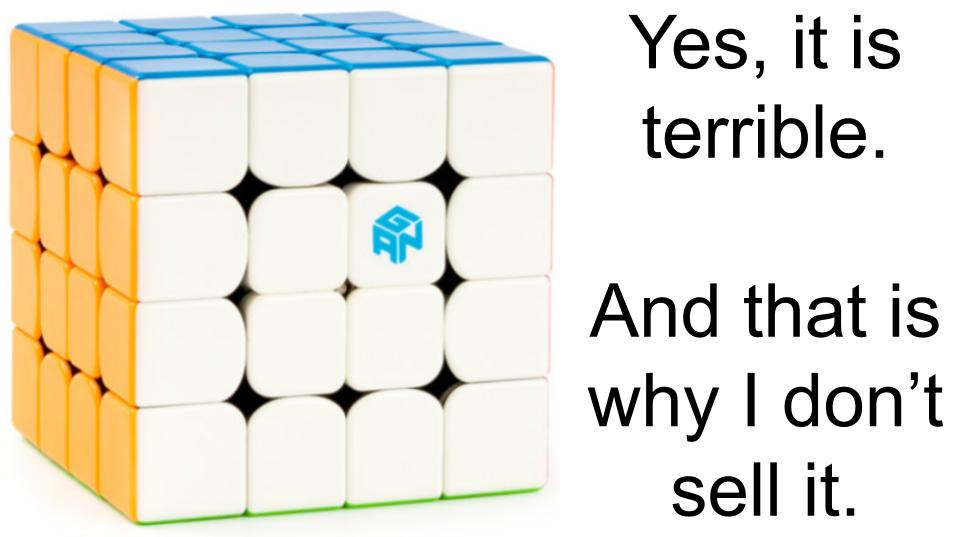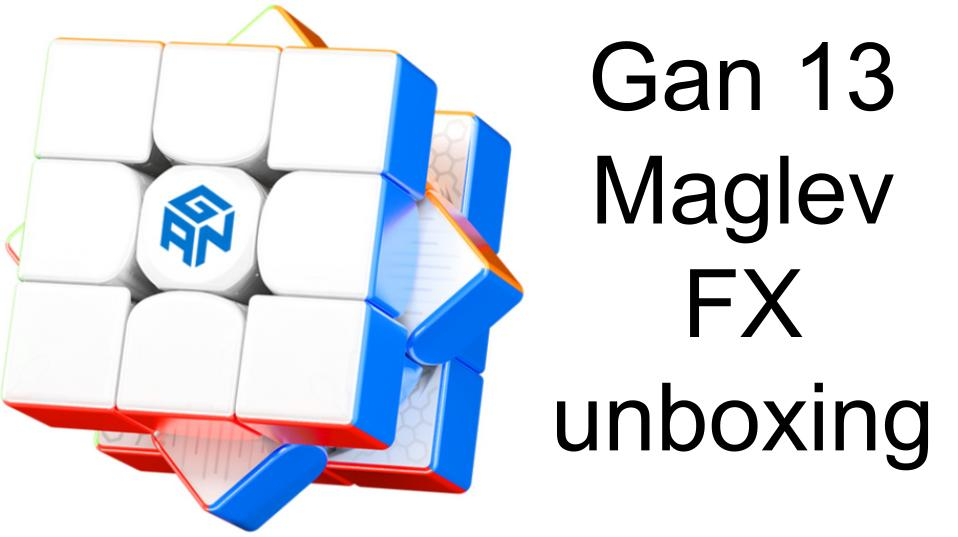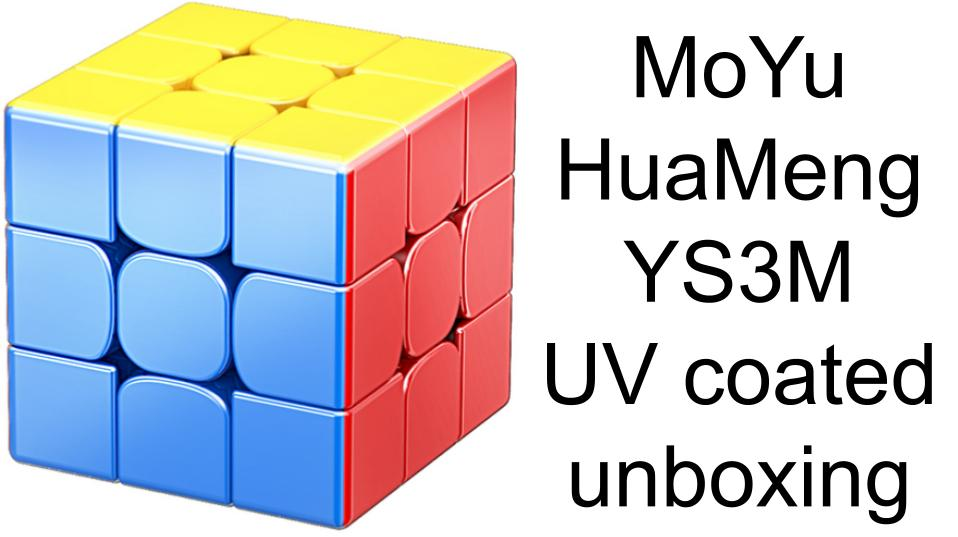# How many combinations are there in a 3x3x3 cube?

The number of combinations in a 3x3x3 is 43,252,003,274,489,856,000, this can be calculated by considering the nature of a 3x3x3 cube:

The centres are all fixed in relation to each other so don't effect the total number of combinations.

A 3x3x3 consists of 12 edges, each have 2 colours on them, these 12 edges can each be in one of 12 locations and can be flipped in two ways, this means that there are 12! possible locations of all the edges, this is 12 factorial which means 12 multiplied by every number below it: 12x11x10x9x8x7x6x5x4x3x2x1 = 479001600. The edges can then be flipped in 2 ways so we can calculated the number of possible cases for this too: 2^12 = 2x2x2x2x2x2x2x2x2x2x2x2 = 4096. So for the edges, the number of combinations is 479001600x4096 = 1961990553600.

a 3x3x3 consists of 8 corners, each have 3 colours on them, these 8 corners can be in one of 8 different locations and can be twisted in 3 ways, this means that there are 8! possible locations for all the corners, 8x7x6x5x4x3x2x1 = 40320. The corners can be twisted in 3 ways so the total number of cases for all these twists is 3^8 = 3x3x3x3x3x3x3x3 = 6561. So the total number of cases for the corners is 40320x6561 = 264539520

For the total number of combinations we can then multiply these two numbers together and get 1961990553600x264539520 = 519024039293878272000. This number is not the actual number of possible scrambles though, this is the total number of possible ways of assembling a 3x3x3 cube, the total number of twists of the corners must be a multiple of 3 for it to be solveable, the total number of edge flips must be a multiple of 2 and swapping only two edges on a 3x3x3 cube is not possible, this means that the number must be divided by 12 to achieve the number of 43,252,003,274,489,856,000, this is the total number of possible combinations that can be reached by conventional means.

I would estimate that the number of 3x3x3 cubes every produced is close to 1 billion, most of these cubes would not be touched much while some would have been solved tens of thousands of times. I estimate that I have done around 150000 solves of a 3x3x3, however, it is only the cross and first 2 F2L pairs that are ever likely to be unique cases, we can assume that my solves and first 20 moves of each scramble may be unique cases which means I may have only ever seen 3 million of those cases! The average cuber has probably done far less solves, however, there are probably millions of cubers who have casually done a few hundred solves in total, I would estimate that the total number of scrambles ever seen by humanity is in the range of 10 trillion, this means that the vast majority have scrambles have never been seen before! If you go on CSTimer and generate a new 3x3x3 scramble, you can say with pretty extreme confidence that that scramble has never been seen by anyone in the world before!

What is even crazier is the fact that it has been proven that every single one of these combinations can be solved in 20 moves or less! Every 3x3x3, all around the world, is 20 moves or less away from being solved!

# Other News##### Gan 460 Unboxing

The Gan 460 is a terrible 4x4x4 speedcube released in 2018, Many people ask...##### Gan 13 Maglev FX unboxing

The Gan 13 Maglev FX is a new speedcube from Gan, it is essentially...##### MoYu HuaMeng YS3M Ball core "Magic Clothes Version" (UV) unboxing

MoYu have now attempted to recreate Gan's classic and popular UV...